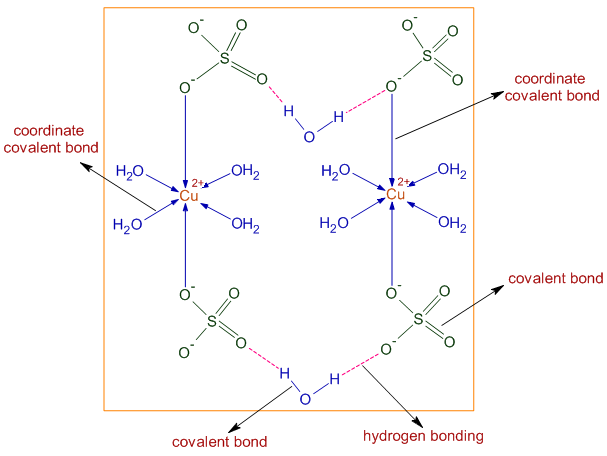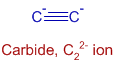Next question >

# TYPES OF CHEMICAL BONDS IIT JEE - NEET

## 1) The types of bonds present in CuSO4.5H2O are only:

(IIT JEE 1983)

1) electrovalent and covalent

2) electrovalent and coordinate covalent

3) electrovalent, covalent  and coordinate covalent

4) covalent  and coordinate covalent

Logic & Solution:

* Structure of CuSO4.5H2O in the solid state is shown below. The Cu2+ ions are attracted towards SO42- ions not only by ionic interactions (electrovalent) but also by coordinate covalent bonds. The Cu2+ ions form coordinate covalent bonds with water as well as sulfate ions.

There are covalent bonds in water and sulfate ions.Conclusion:

The correct option is 3.

Note:

There is also hydrogen bonding between water and sulfate ions. Only four water molecules are forming dative bonds with Cu2+. The fifth molecules is involved in H-bonding.

## Related questions

### 2) Which of the following contains both polar and non-polar bonds?

(IIT JEE 1997)

1) NH4Cl

2) HCN

3) H2O2

4) CH4

Logic & solution:

1) In NH4Cl, the N-H bond is polar due to appreciable electronegativity difference between N (3.0) and H (2.1).

2) In HCN, the H-C and C-N bonds are polar due to electronegativity difference between C (2.5) and N (3.0) for H-C bond as well as between C (2.5) and  H (2.1) for C-N bond.

3) In H2O2, the O-H bond is polar due to electronegativity difference between O (3.5) and H (2.1). Whereas, O-O bond is non-polar.

4) In CH4, the C-H bonds are also polar.Note: Even though, the C-H bonds have a little polarity, you might have seen that they are considered to be non-polar in the study of organic chemistry. It is a relative thing. They are less polar when compared to bonds formed by carbon with other atoms like: C-O, C-N, C-X etc. Hence C-H bonds are treated as non-polar.

But according to the tone of this question, C-H bonds are taken as polar since there is electronegativity difference.

Conclusion:

Option '3' is correct.

### 3) The number and type of bonds between two carbon atoms in CaC2 are:

(IIT JEE 1996)

1) one σ and one π bonds

2) one σ and two π bonds

3) one σ and one and half  π bonds

4) one σ bond

Logic & solution:

CaC2 can be divided into Ca2+ and C22- ions. The structure of C22- ion is shown below:There is a triple bond between two carbons. Among three bonds, only one is sigma bond and the remaining two are pi bonds.

Conclusion:

Option '2' is correct.

### 4) Which one of the following molecule contains both ionic and covalent bonds?

(EAMCET 2002-E)

1) CH2Cl2

2) K2SO4

3) BeCl2

4) SO2

Logic & solution:

Only in K2SO4, there are ionic as well as covalent bonds. The K+ and SO42- ions are attracted by electrostatic forces. Sulfur atom has covalent bonds in sulfate ion.Conclusion:

Option '2' is correct.

### 5) Element 'X' is strongly electropositive and element 'Y' is strongly electronegative. Both are univalent. The compound formed will be:

(IIT JEE 1980)

1) X+Y-

2) Y+X-

3) X-Y

4) X→Y

Logic & solution:

Strongly electropositive elements tend to lose electrons due to relatively low ionization potentials to form cations (like X+) and strongly electronegative elements tend to gain electrons to form anions (like Y-). Hence the bond formed between them is predominantly ionic in nature.

Conclusion:

Option '1' is correct.

### 6) The bond between two identical non metal atoms has a pair of electrons. The correct statement about this pair is:

(IIT JEE 1986)

1) Unequally shared between the two.

2) Transferred fully from one atom to another

3) Has identical spins.

4) Equally shared between the atoms.

Logic & solution:

Identical atoms possess same electronegativity values and hence share the electron pair equally by forming a non polar covalent bond.

Conclusion:

Option '4' is correct.

### 7) The bonds present in N2O5 are:

(IIT JEE 1986)

1) only ionic

2) covalent and coordinate

3) only covalent

4) covalent and ionic

Logic & solution:

Since nitrogen and oxygen are non metals, the bonds between them are NOT ionic.

The structure of N2O5 is given below. There are both covalent and coordinate covalent bonds.Conclusion:

Option '2' is correct.

### Homework

1) ?

 Next question >
Author: Aditya vardhan Vutturi